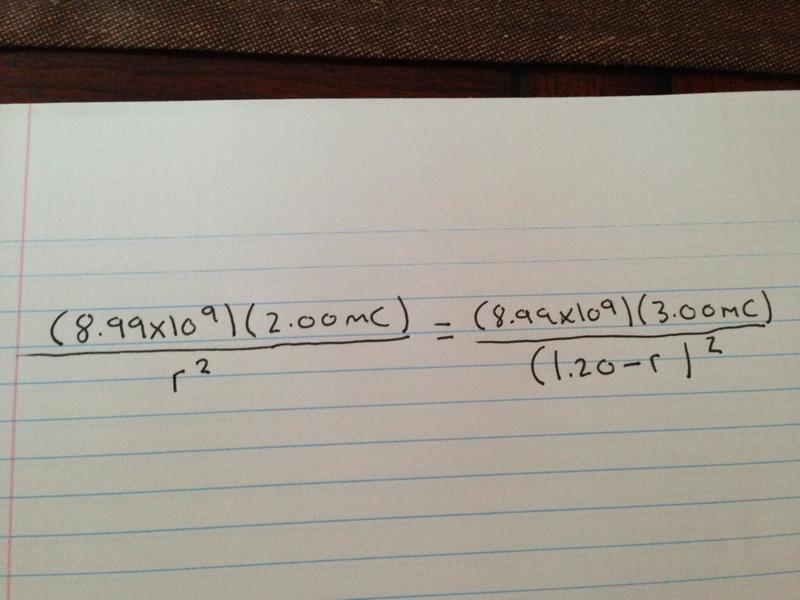Determining point where net electric field is zero

Question
A negative charge of 3.00 mC is 1.20m to the right of another negative charge of 2.00 mC. Calculate the point along the same line between the two charges where the net electric field will be zero.

Formulas
E=kq/r2

Attempt at Solving
Well, I get as far as setting E1=kq/r2 equal to E2=kq/r2 but am at lost as to what to do next. I tried bringing the equations all to one side and setting them equal to zero but can't seem to isolate for r. I also tried cross multiplying and dividing but didn't get the answer from that either. I know the answer (0.661m left of the -3.00 charge) but am unable to find out how to get the answer. If someone could provide a detailed, comprehensive explanation as to how to figure this question out it would be greatly appreciated.

Cheers!

As stated in the problem, the distance between two charges is 1.2 m, and you know that there is a point somewhere in between these two where electric field is zero. Imagine that point is p, as is (x) m away from -2 mC charge and (1.2 - x) m away from -3 mC charge.
it would look like sth like this: -2mC ----- P ----------- -3mc

As stated in the problem, the distance between two charges is 1.2 m, and you know that there is a point somewhere in between these two where electric field is zero. Imagine that point is p, as is (x) m away from -2 mC charge and (1.2 - x) m away from -3 mC charge.
it would look like sth like this: -2mC ----- P ----------- -3mc

Like This?Like This? If so how do I isolate for r?yes! exactly. just notice that the r we find is the distance from -2 mC charge! (because that's how we defined it, you can just subtract it from 1.2 to find the distance from -3 mC if you want)

the K in both sides cancel out each other, you can bring r's to one side of the equation r^2 / (1.2 -r)^2 and 2/3 to the other. from there on it's just some algebra. (it's best to take the square root of both sides to get ride of r's power of 2)

Ok great!.....however I am having trouble isolating for r....On one side I have r/(1.20-r) and on the other side I have square root of 2.00/3.00 (due to squaring both sides to get rid of the power of 2. How do I isolate for this r?

Square root of 2/3 is roughly, 0.816. substitute this. now you have r/(1.2-r) = 0.816 You should have studied solving of these equation in algebra. anyway, you can multiply both side by (1.2-r) which results in r = 0.816(1.2-r). Multiply the right side: r = 0.979 - 0.816 r
from here it's really easy.